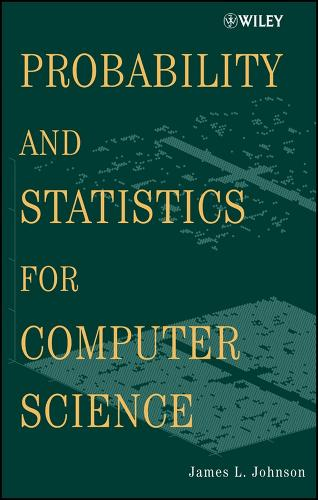•# Probability and Statistics for Computer Science (Hardback)

(author)
£212.00
Hardback 760 Pages / Published: 30/07/2003
• We can order this

Usually dispatched within 3 weeks

Comprehensive and thorough development of both probability and statistics for serious computer scientists; goal-oriented: "to present the mathematical analysis underlying probability results" Special emphases on simulation and discrete decision theory Mathematically-rich, but self-contained text, at a gentle pace Review of calculus and linear algebra in an appendix Mathematical interludes (in each chapter) which examine mathematical techniques in the context of probabilistic or statistical importance Numerous section exercises, summaries, historical notes, and Further Readings for reinforcement of content

Publisher: John Wiley & Sons Inc
ISBN: 9780471326724
Number of pages: 760
Weight: 1264 g
Dimensions: 242 x 166 x 44 mm